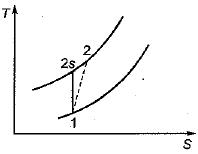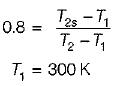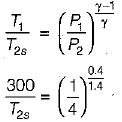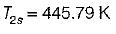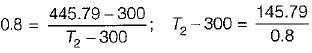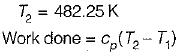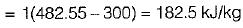Courses

# Test: Compressors - 3

## 20 Questions MCQ Test Topicwise Question Bank for Mechanical Engineering | Test: Compressors - 3

Description
This mock test of Test: Compressors - 3 for Mechanical Engineering helps you for every Mechanical Engineering entrance exam. This contains 20 Multiple Choice Questions for Mechanical Engineering Test: Compressors - 3 (mcq) to study with solutions a complete question bank. The solved questions answers in this Test: Compressors - 3 quiz give you a good mix of easy questions and tough questions. Mechanical Engineering students definitely take this Test: Compressors - 3 exercise for a better result in the exam. You can find other Test: Compressors - 3 extra questions, long questions & short questions for Mechanical Engineering on EduRev as well by searching above.
QUESTION: 1

Solution:
QUESTION: 2

### A large clearance volume in reciprocating compressor results in

Solution:

The clearance volume is the space between piston top and inner surface of the cylinder head. The clearance volume reduces the capacity of the compressor and volume flow rate.

QUESTION: 3

### If n is the polytropic index of compression and p2/p1 is the pressure ratio for a three-stage compressor with ideal intercooling, the expression for total work of three stage is

Solution:
QUESTION: 4

A P-v diagram has been obtained from a test on a reciprocating compressor. Which of the following represents that diagram?

Solution:
QUESTION: 5

When is the work required for compression of an ideal gas between two pressures in a reciprocating compressor minimum?

Solution:
QUESTION: 6

Performance of a reciprocating compressor is expressed by

Solution:

Isothermal efficiency of reciprocating compressor is defined as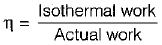QUESTION: 7

Which one of the following type of compressor is mostly used for supercharging of I.C. engine

Solution:

Since very small pressure ratio is required in supercharging of I.C. engine root blower or centrifugal compressor is used.

QUESTION: 8

Rotary compressor is best suited for

Solution:

Rotary compressor is suitable for low pressure ratio and large quantity of air.

QUESTION: 9

Roots blower is an example of

Solution:
QUESTION: 10

Centrifugal compressors are suitable for large discharge and wider mass flow range, but at a relatively low discharge pressure of the order of 10 bars, because of

Solution:
QUESTION: 11

In a centrifugal compressor assuming the same overall dimensions, blade inlet angle and rotational speeds, which of the following bladings will given the maximum pressure rise?

Solution:

In centrifugal compressors, forward blading is used for higher pressure ratio, while backward blading have uniform performance over wide range of working conditions.

QUESTION: 12

The usual assumption in elementary compressor cascade theory is that

Solution:
QUESTION: 13

What, docs application of centrifugal air compressors lead to?

Solution:

Centrifugal air compressor increases the frontal area of air craft which increases drag. So axial flow compressor are used.

QUESTION: 14

Degree of reaction in an axial flow compressor is defined as the ratio of static enthalpy rise in the

Solution:

Degree of reaction is the ratio of static enthalpy rise in rotor to static enthalpy rise in the stage.

QUESTION: 15

Surging basically implies

Solution:

Surging is associated with a sudden drop in pressure on delivery side of impeller with violent aerodynamic pulsation which is transmitted throughout the whole machine.

QUESTION: 16

Phenomenon of choking in compressor means

Solution:

Chocking mean maximum mass flow rate which is not dependent on pressure ratio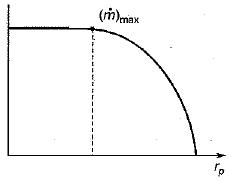QUESTION: 17

The flow in the vaneless space between the impeller exit and diffuser inlet of a centrifugal compressor can be assumed as

Solution:

There is clearance between the impeller and vane leading edge, called vaneless space. In the vaneless space, the flow follow an approximately logarithmic spiral path to leading edge of the vanes after which it is constrained by the diffuser channel to follow the blade shape curvature.

QUESTION: 18

The optimum intermediate pressure p1 for a gas turbine plant operating between pressure limits p1 and p2 with perfect intercooling between the two stages of compression (with identical isentropic efficiency) is given by

Solution:
QUESTION: 19

Consider a two stage reciprocating air compressor with a perfect intercooler operating at the best intermediate pressure. Air enters the low pressure cylinder at 1 bar, 27°C and leaves the high pressure cylinder at 9 bar. Assume the index of compression and expansion in each stage is 1.4, and that for air R - 286.7 J/kg K, the work done per kg air in the high pressure cylinder is [Take 30.286 = 1.37]

Solution: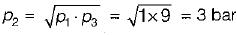Work done in either cylinder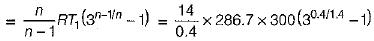= 3.5 x 286.7 x 300 (1.37 - 1) = 111 kJ

QUESTION: 20

Air (cp = 1 kJ/kgK, γ - 1.4) enters a compressor at a temperature of 27°C. The compressor pressure ratio is 4. Assuming an efficiency of 80%, the compressor work required in kJ/kg is [Take 0.250.286 = 0.67]

Solution: